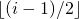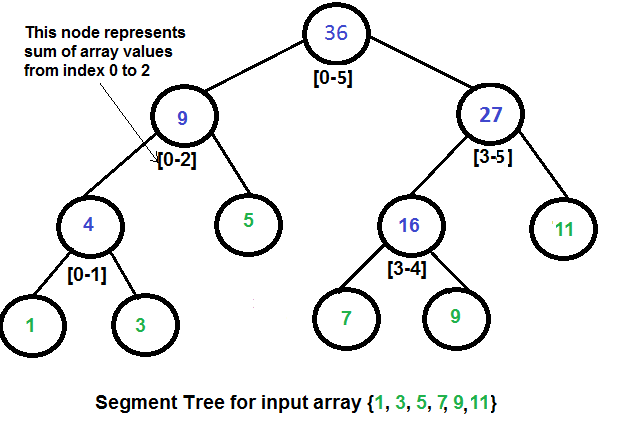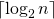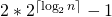Segment Tree (Sum of given range)

Let us consider the following problem to understand Segment Trees.

We have an array arr[0 . . . n-1]. We should be able to
1 Find the sum of elements from index l to r where 0 <= l <= r <= n-1

2 Change value of a specified element of the array to a new value x. We need to do arr[i] = x where 0 <= i <= n-1.

simple solution is to run a loop from l to r and calculate the sum of elements in the given range. To update a value, simply do arr[i] = x. The first operation takes O(n) time and the second operation takes O(1) time.

Another solution is to create another array and store sum from start to i at the ith index in this array. The sum of a given range can now be calculated in O(1) time, but update operation takes O(n) time now. This works well if the number of query operations is large and very few updates.

What if the number of query and updates are equal? Can we perform both the operations in O(log n) time once given the array? We can use a Segment Tree to do both operations in O(Logn) time.

Representation of Segment trees
1. Leaf Nodes are the elements of the input array.
2. Each internal node represents some merging of the leaf nodes. The merging may be different for different problems. For this problem, merging is sum of leaves under a node.

An array representation of tree is used to represent Segment Trees. For each node at index i, the left child is at index 2*i+1, right child at 2*i+2 and the parent is at.How does above segment tree look in memory?
Like Heap, the segment tree is also represented as an array. The difference here is, it is not a complete binary tree. It is rather a full binary tree (every node has 0 or 2 children) and all levels are filled except possibly the last level. Unlike Heap, the last level may have gaps between nodes. Below are the values in the segment tree array for the above diagram.

Below is memory representation of segment tree for input array {1, 3, 5, 7, 9, 11}
st[] = {36, 9, 27, 4, 5, 16, 11, 1, 3, DUMMY, DUMMY, 7, 9, DUMMY, DUMMY}

The dummy values are never accessed and have no use. This is some wastage of space due to simple array representation. We may optimize this wastage using some clever implementations, but code for sum and update becomes more complex.

Construction of Segment Tree from given array
We start with a segment arr[0 . . . n-1]. and every time we divide the current segment into two halves(if it has not yet become a segment of length 1), and then call the same procedure on both halves, and for each such segment, we store the sum in the corresponding node.
All levels of the constructed segment tree will be completely filled except the last level. Also, the tree will be a Full Binary Tree because we always divide segments in two halves at every level. Since the constructed tree is always a full binary tree with n leaves, there will be n-1 internal nodes. So the total number of nodes will be 2*n-1. Note that this does not include dummy nodes.

What is the total size of the array representing segment tree?

If n is a power of 2, then there are no dummy nodes. So the size of the segment tree is 2n-1 (n leaf nodes and n-1) internal nodes. If n is not a power of 2, then the size of the tree will be 2*x-1 where x is the smallest power of 2 greater than n. For example, when n = 10, then size of array representing segment tree is 2*16-1 = 31.
An alternate explanation for size is based on heignt. Height of the segment tree will be. Since the tree is represented using array and relation between parent and child indexes must be maintained, size of memory allocated for segment tree will be.

Query for Sum of given range
Once the tree is constructed, how to get the sum using the constructed segment tree. The following is the algorithm to get the sum of elements.

int getSum(node, l, r)
{
if the range of the node is within l and r
return value in the node
else if the range of the node is completely outside l and r
return 0
else
return getSum(node's left child, l, r) +
getSum(node's right child, l, r)
}

Update a value
Like tree construction and query operations, the update can also be done recursively. We are given an index which needs to be updated. Let diff be the value to be added. We start from the root of the segment tree and add diff to all nodes which have given index in their range. If a node doesnt have a given index in its range, we dont make any changes to that node.

Implementation:
Following is the implementation of segment tree. The program implements construction of segment tree for any given array. It also implements query and update operations.

// C++ program to show segment tree operations like construction, query
// and update
#include <bits/stdc++.h>
using namespace std;

// A utility function to get the middle index from corner indexes.
int getMid(int s, int e) { return s + (e -s)/2; }

/* A recursive function to get the sum of values in the given range
of the array. The following are parameters for this function.

st --> Pointer to segment tree
si --> Index of current node in the segment tree. Initially
0 is passed as root is always at index 0
ss & se --> Starting and ending indexes of the segment represented
by current node, i.e., st[si]
qs & qe --> Starting and ending indexes of query range */
int getSumUtil(int *st, int ss, int se, int qs, int qe, int si)
{
// If segment of this node is a part of given range, then return
// the sum of the segment
if (qs <= ss && qe >= se)
return st[si];

// If segment of this node is outside the given range
if (se < qs || ss > qe)
return 0;

// If a part of this segment overlaps with the given range
int mid = getMid(ss, se);
return getSumUtil(st, ss, mid, qs, qe, 2*si+1) +
getSumUtil(st, mid+1, se, qs, qe, 2*si+2);
}

/* A recursive function to update the nodes which have the given
index in their range. The following are parameters
st, si, ss and se are same as getSumUtil()
i --> index of the element to be updated. This index is
in the input array.
diff --> Value to be added to all nodes which have i in range */
void updateValueUtil(int *st, int ss, int se, int i, int diff, int si)
{
// Base Case: If the input index lies outside the range of
// this segment
if (i < ss || i > se)
return;

// If the input index is in range of this node, then update
// the value of the node and its children
st[si] = st[si] + diff;
if (se != ss)
{
int mid = getMid(ss, se);
updateValueUtil(st, ss, mid, i, diff, 2*si + 1);
updateValueUtil(st, mid+1, se, i, diff, 2*si + 2);
}
}

// The function to update a value in input array and segment tree.
// It uses updateValueUtil() to update the value in segment tree
void updateValue(int arr[], int *st, int n, int i, int new_val)
{
// Check for erroneous input index
if (i < 0 || i > n-1)
{
cout<<"Invalid Input";
return;
}

// Get the difference between new value and old value
int diff = new_val - arr[i];

// Update the value in array
arr[i] = new_val;

// Update the values of nodes in segment tree
updateValueUtil(st, 0, n-1, i, diff, 0);
}

// Return sum of elements in range from index qs (quey start)
// to qe (query end). It mainly uses getSumUtil()
int getSum(int *st, int n, int qs, int qe)
{
// Check for erroneous input values
if (qs < 0 || qe > n-1 || qs > qe)
{
cout<<"Invalid Input";
return -1;
}

return getSumUtil(st, 0, n-1, qs, qe, 0);
}

// A recursive function that constructs Segment Tree for array[ss..se].
// si is index of current node in segment tree st
int constructSTUtil(int arr[], int ss, int se, int *st, int si)
{
// If there is one element in array, store it in current node of
// segment tree and return
if (ss == se)
{
st[si] = arr[ss];
return arr[ss];
}

// If there are more than one elements, then recur for left and
// right subtrees and store the sum of values in this node
int mid = getMid(ss, se);
st[si] = constructSTUtil(arr, ss, mid, st, si*2+1) +
constructSTUtil(arr, mid+1, se, st, si*2+2);
return st[si];
}

/* Function to construct segment tree from given array. This function
allocates memory for segment tree and calls constructSTUtil() to
fill the allocated memory */
int *constructST(int arr[], int n)
{
// Allocate memory for the segment tree

//Height of segment tree
int x = (int)(ceil(log2(n)));

//Maximum size of segment tree
int max_size = 2*(int)pow(2, x) - 1;

// Allocate memory
int *st = new int[max_size];

// Fill the allocated memory st
constructSTUtil(arr, 0, n-1, st, 0);

// Return the constructed segment tree
return st;
}

// Driver program to test above functions
int main()
{
int arr[] = {1, 3, 5, 7, 9, 11};
int n = sizeof(arr)/sizeof(arr);

// Build segment tree from given array
int *st = constructST(arr, n);

// Print sum of values in array from index 1 to 3
cout<<"Sum of values in given range = "<<getSum(st, n, 1, 3)<<endl;

// Update: set arr = 10 and update corresponding
// segment tree nodes
updateValue(arr, st, n, 1, 10);

// Find sum after the value is updated
cout<<"Updated sum of values in given range = "
<<getSum(st, n, 1, 3)<<endl;
return 0;
}

Output:

Sum of values in given range = 15
Updated sum of values in given range = 22

Time Complexity:
Time Complexity for tree construction is O(n). There are total 2n-1 nodes, and value of every node is calculated only once in tree construction.

Time complexity to query is O(Logn). To query a sum, we process at most four nodes at every level and number of levels is O(Logn).

The time complexity of update is also O(Logn). To update a leaf value, we process one node at every level and number of levels is O(Logn).More Articles of M Mounika:

Name Views Likes
C++ Inplace Rotate square matrix by 90 degrees 561 0
C++ Introduction to Greedy Approach 1635 2
C++ Gold Mine Problem 593 0
C++ Anagram substring Search 243 0
C++ Segment Trees 525 2
Disjoint Data Structures 336 1
C++ Greedy Approach-Activity Selection Problem 1830 1
C++ Trie Datastructure 1147 1
C++ Minimum Removal of Characters from string to make its permutation as palindrome 303 0
C++ Greedy Approach Minimum number of coins 1180 0
C++ Greedy Approach-Huffman Codes 3543 1
C++ Manachars Algorithm 528 0
C++ smallest substring with maximum distinct characters 888 0
C++ Trie Cost of Data 712 1
C++ Greedy Approach Maximum product Subset 760 1
C++ Greedy Approach Maximum height Pyramid 687 1
C++ Greedy Approach-Egyptian Fractions 1092 0
C++ String Matching(KMP Algorithm) 520 0
C++ K Centers Problem 666 0
C++ Find Non Repeating Elements in Array 719 0
C++ Greedy Approach Minimum Product Subset 791 1
C++ Merge K Sorted Arrays 300 0
C++ Number of Bracket Reversals needed to make expression Balanced 169 0
C++ Basics of String Manipulation 233 1
C++ Problem On Disjoint Data Structures 294 0
C++ Find Nth Catalan Number 446 0
C++ Greedy Approach String lexicographically largest subsequence 673 0
C++ Merge Two Binary Trees 449 0
C++ Remove Duplicates From String 2038 0
C++ Minimum characters added at front of string in palindrome conversion 249 0
Disjoint Data Structures Part2 260 0
C++ Greedy Approach Prims MST 739 1
C++ Greedy Approach N-array maximum sum 569 1
C++ String Matching(Naive Algorithm) 514 0
C++ Flood Fill Algorithm 996 0
C++ WildCard pattern matching 607 0
C++ String Matching(Z Algorithm) 275 0
C++ Meta Strings 397 0
C++ Sum of Numbers From Root To Leaf Paths 447 0
C++ Greedy Approach Lexicographically largest subsequence 652 0
C++ Disjoint Data Structures Part3 218 0
C++ Sieve Of Erastosthenes 342 0
C++ String to Palindrome with Append Function 328 0
C++ Search String in Grid 782 0
C++ Greedy Approach Policemen Catch Thieves 1111 1
C++ Smallest window with all characters in string 630 0
C++ Greedy Approach Fractional Knapsack 2045 1
C++ Disjoint Data Structure Cycle Detection 269 0
C++ Segmented Sieve (Print Primes In a Range) 807 0
C++ Greedy Approach Krushkals MST 711 1